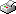Print This Page Daniel Bruce -- licentiate thesis -- A Model for Bivariate Bernoulli Variables - Optimal Design and Inference Summary This thesis presents a model for dependent and equally distributed Bernoulli variables. The model, which incorporates explanatory variables, is a multivariate generalized linear models (MGLM). In the particular case with just two dependent variables, the model can be further divided into three different cases according to symmetry properties and independency between the variables. Different cases can be distinguished using different parameter restrictions. Locally D-optimal designs are given for each case. The special case with symmetry properties for two dependent variables is examined in detail. D-optimal designs for the special case have 2, 3, or 4 design points, where the number of design points are determined by the log-odds ratio. The log-odds ratio also partly explain the appearance of the probability distribution of the response variables. For some 2-point designs and some 4-point designs a general expression for D-optimal designs is given. These proposed designs are found to have a high efficiency. In case the variables are independent, a general expression for D-optimal designs is derived. Score tests and likelihood ratio tests are derived for testing if the Bernoulli variables are independent. Test statistics for two particular situations are outlined in detail. Numerical illustrations of these test statistics are presented in three examples, including one with visual impairment data. An optimal design for maximization of the local asymptotic power of the score test is proposed. The asymptotically power function based on the proposed design is compared with power function for finite sample sizes using a small simulation experiment. The finite sample power of the proposed design resembles the properties of the asymptotic power as long as the log-odds ratio is negative. The simulation study also indicate that there can be problems when computing the test statistic for large values of the log-odds ratio. Since the locally optimal design depends on the unknown parameters, the robustness of the design is examined. The examination shows that the optimal design is robust against fairly incorrect parameter values.